Line chart in Matplotlib – Python

• Last Updated : 20 Oct, 2020

Matplotlib is a data visualization library in Python. The pyplot, a sublibrary of matplotlib, is a collection of functions that helps in creating a variety of charts.  Line charts are used to represent the relation between two data X and Y on a different axis. Here we will see some of the examples of a line chart in Python :

Simple line plots

First import Matplotlib.pyplot library for plotting functions. Also, import the Numpy library as per requirement. Then define data values x and y.

Python3

 # importing the required librariesimport matplotlib.pyplot as pltimport numpy as np  # define data valuesx = np.array([1, 2, 3, 4])  # X-axis pointsy = x*2  # Y-axis points  plt.plot(x, y)  # Plot the chartplt.show()  # display

Output: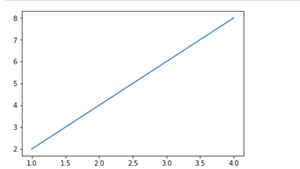Simple line plot between X and Y data

we can see in the above output image that there is no label on the x-axis and y-axis. Since labeling is necessary for understanding the chart dimensions. In the following example, we will see how to add labels, Ident in the charts

Python3

 import matplotlib.pyplot as pltimport numpy as np    # Define X and Y variable datax = np.array([1, 2, 3, 4])y = x*2  plt.plot(x, y)plt.xlabel("X-axis")  # add X-axis labelplt.ylabel("Y-axis")  # add Y-axis labelplt.title("Any suitable title")  # add titleplt.show()

Output: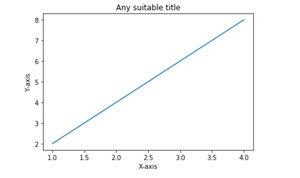Simple line plot with labels and title

Multiple charts

We can display more than one chart in the same container by using pyplot.figure() function. This will help us in comparing the different charts and also control the look and feel of charts .

Python3

 import matplotlib.pyplot as pltimport numpy as np    x = np.array([1, 2, 3, 4])y = x*2  plt.plot(x, y)plt.xlabel("X-axis")plt.ylabel("Y-axis")plt.title("Any suitable title")plt.show()  # show first chart  # The figure() function helps in creating a# new figure that can hold a new chart in it.plt.figure()x1 = [2, 4, 6, 8]y1 = [3, 5, 7, 9]plt.plot(x1, y1, '-.')  # Show another chart with '-' dotted lineplt.show()

Output: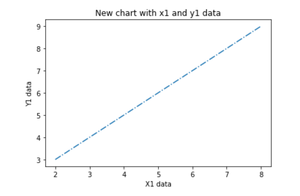Multiple plots on the same axis

Here we will see how to add 2 plots within the same axis.

Python3

 import matplotlib.pyplot as pltimport numpy as np  x = np.array([1, 2, 3, 4])y = x*2  # first plot with X and Y dataplt.plot(x, y)  x1 = [2, 4, 6, 8]y1 = [3, 5, 7, 9]  # second plot with x1 and y1 dataplt.plot(x1, y1, '-.')  plt.xlabel("X-axis data")plt.ylabel("Y-axis data")plt.title('multiple plots')plt.show()

Output: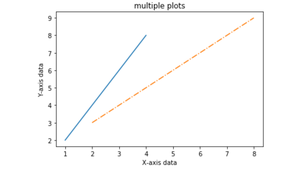Fill the area between two plots

Using the pyplot.fill_between() function we can fill in the region between two line plots in the same graph. This will help us in understanding the margin of data between two line plots based on certain conditions.

Python3

 import matplotlib.pyplot as pltimport numpy as np  x = np.array([1, 2, 3, 4])y = x*2  plt.plot(x, y)  x1 = [2, 4, 6, 8]y1 = [3, 5, 7, 9]  plt.plot(x, y1, '-.')plt.xlabel("X-axis data")plt.ylabel("Y-axis data")plt.title('multiple plots')  plt.fill_between(x, y, y1, color='green', alpha=0.5)plt.show()

Output: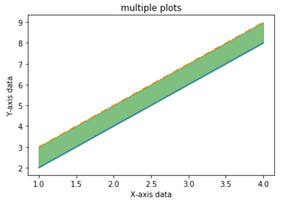Fill the area between Y and Y1 data corresponding to X-axis data

My Personal Notes arrow_drop_up